﻿ 侧向风下航母甲板风涡流场的模拟研究
 舰船科学技术2017, Vol. 39Issue (1): 45-50PDF

Simulated study to wind deck vortex flow of aircraft carriers under cross wind
YUAN Shu-sheng, NIAN Song-lei, ZHAO Yuan-li
Naval Aeronautical and Astronautical University, Yantai 264001, China
Abstract: The control equations with the hypothesis of low Mach air flow and the large eddy simulation method of turbulent flows are used to study the characteristics of vortex in the wind over deck of aircraft carriers under the condition of cross wind. The time-averaged value, the variety scope and the variety period of pressures in the region over the former deck are near invariable. The time-averaged value, the variety scope and the variety period of pressures in the region over the tail deck are little larger than that at the former deck.
Key words: aircraft carrier     wind over deck     large eddy simulation     vortex flow

1 大涡模拟控制方程组

 $\bar p\left( {{x},t} \right) = {\bar p_0}\left( z \right) + \tilde p\left( {{x},t} \right),$ (1)

 ${T_0}\left( z \right) = {T_\infty } + \Gamma z,$ (2)
 ${\bar p_0}\left( z \right) = {p_\infty }{\left( {\frac{{{T_\infty } + \Gamma z}}{{{T_\infty }}}} \right)^{g/R\Gamma }。}$ (3)

 ${\bar p_0} = {\rho _0}R{T_0}。$ (4)

 $\frac{{\partial \bar \rho }}{{\partial t}} + \nabla \cdot \bar \rho {\tilde u} = 0,$ (5)
 $\bar \rho \left( {\frac{{\partial {\tilde u}}}{{\partial t}} + \nabla (\frac{{{{\left| {{\tilde u}} \right|}^2}}}{2} + \frac{{\tilde p}}{{\bar \rho }})} \right) + \nabla {\bar p_0} = \bar \rho {g} + \nabla \cdot {{\bar \tau }_l} + \nabla \cdot {\tau },$ (6)
 $\frac{\partial }{{\partial t}}(\bar \rho {C_p}\tilde T) + \nabla \cdot (\bar \rho {\tilde u}{C_p}\tilde T) = \frac{{D{{\bar p}_0}}}{{Dt}} + \nabla \cdot (\lambda \nabla \tilde T) + \nabla \cdot {q},$ (7)

 $\frac{{\partial {\tilde u}}}{{\partial t}}\!=\!{\tilde u}\!\times\!{\tilde \omega }\!+\!\frac{1}{{\bar \rho }}[(\bar \rho\!-\!{\rho _0}){\rm{g}}\!+\!\nabla \cdot {{\bar \tau }_l}\!+\!\nabla \cdot {\tau }]\!+\!\tilde p\nabla \left( {\frac{1}{{\bar \rho }}} \right)\!-\!\nabla P,$ (8)
 $\begin{split} \\[-12pt] \nabla \cdot {\tilde u} =& \frac{1}{{\bar \rho {C_p}\tilde T}}\left[ {\nabla \cdot (\lambda \nabla \tilde T) + \nabla \cdot {q}- {\tilde u} \cdot \nabla \left( {\bar \rho {C_p}\tilde T} \right)} \right] + \\ & \left( {\frac{1}{{{{\bar p}_0}}}- \frac{1}{{\bar \rho {C_p}\tilde T}}} \right)w{\rho _0}g, \end{split}$ (9)
 $\begin{split} \\[-12pt] & {\nabla ^2}P =- \frac{\partial }{{\partial t}}\left( {\nabla \cdot {\tilde u}} \right) +\nabla \cdot\\ & \;\;\;\;\;\;\;\;\;\;\left\{ {{\tilde u} \times {\tilde \omega }\!+\!\frac{1}{{\bar \rho }}[(\bar \rho\!-\!{\rho _0}){\rm{g}}\!+\!\nabla \cdot {{\bar \tau }_l}\!+\!\nabla \cdot \tau ]\!+\!\tilde p\nabla \left( {\frac{1}{{\bar \rho }}} \right)} \right\}。 \end{split}$ (10)

2 模拟对象与工况参数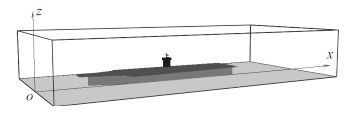图 1 计算区域与航母模型示意图 Fig. 1 The schematic diagram for calculation area and aircraft carrier

3 结果分析与讨论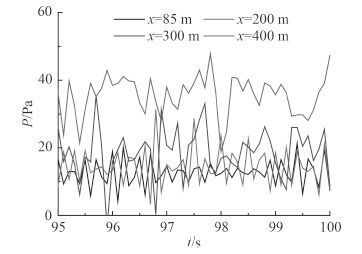图 2 侧向风条件下航母甲板上方不同位置处压强随着时间变化的模拟结果 Fig. 2 The simulation results of the pressure changing over time under lateral wind conditions at different location of the upper deck of the carrier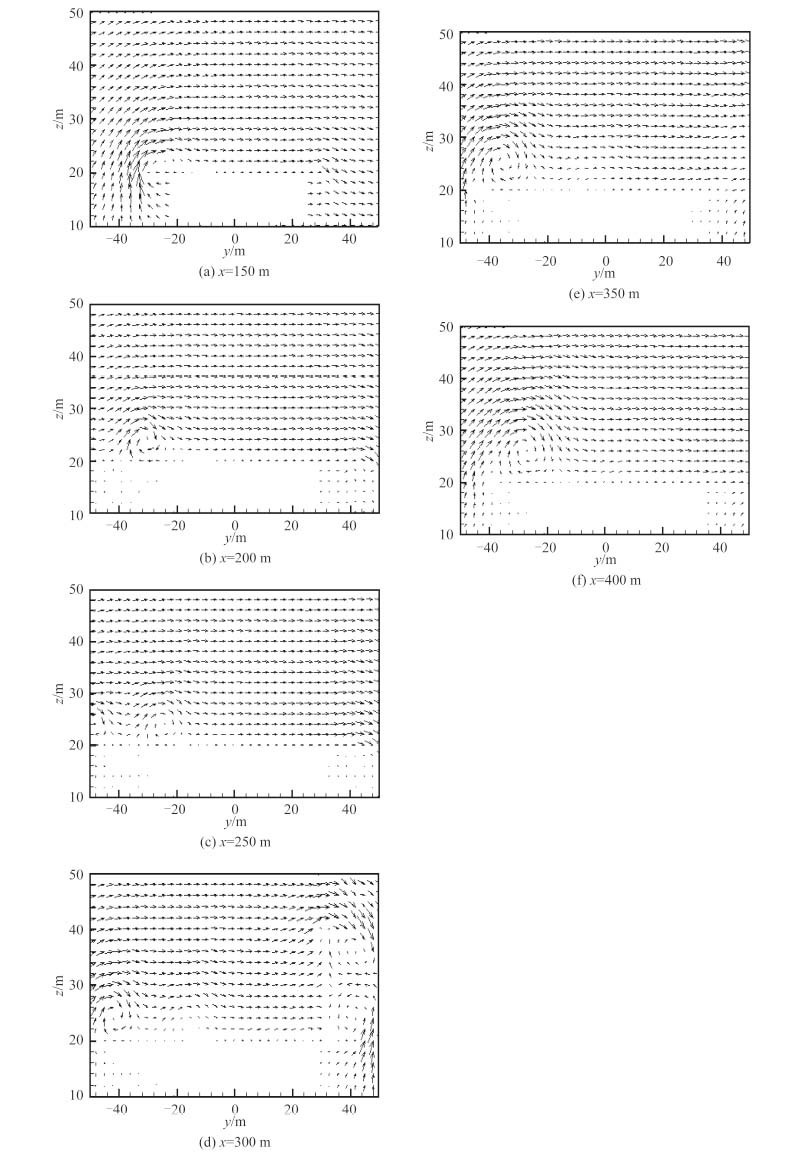图 3 甲板风时均稳定后，距舰艏不同距离垂直截面上时均速度分布 Fig. 3 After the deck wind time average stabilization, time-averaged velocity distribution on the vertical section of different distance from the ship bow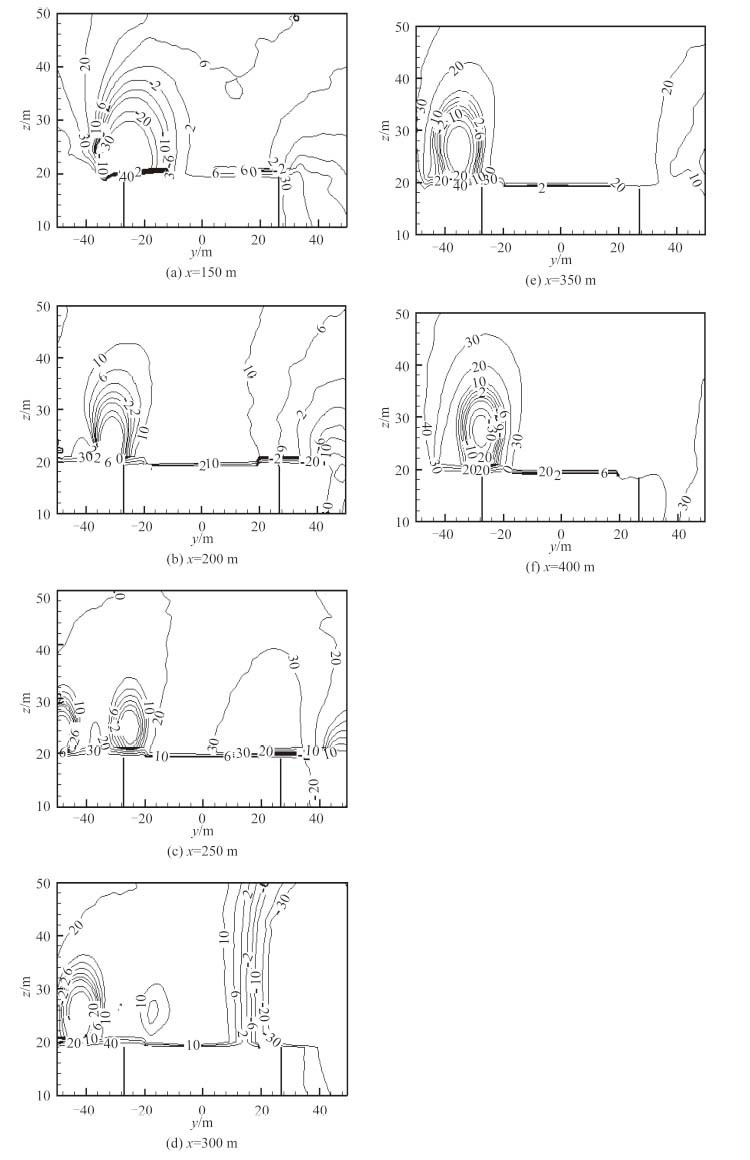图 4 甲板风时均稳定后，距舰艏不同距离垂直截面上时均压强（Pa）分布 Fig. 4 After the deck wind time average stabilization, time-averaged pressure distribution on the vertical section of different distance from the ship bow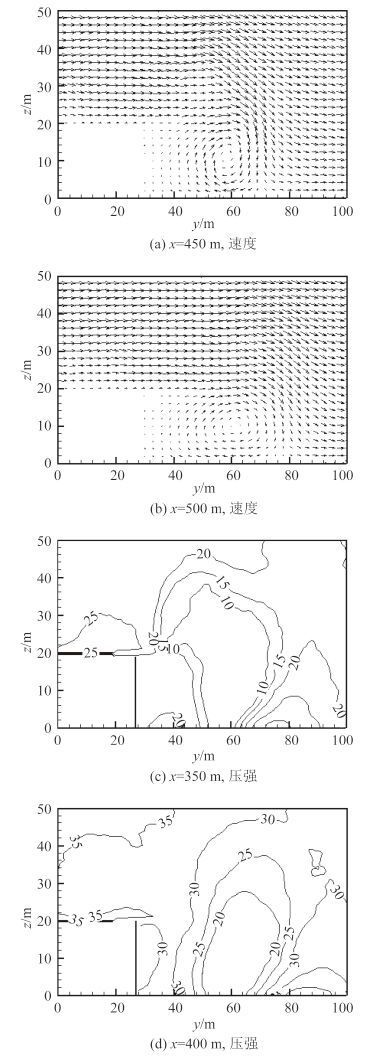图 5 x = 350 m 和x = 400 m 垂直截面上背风侧的时均速度和时均压强（Pa）分布 Fig. 5 Underx = 350 m andx = 400 m, time-averaged velocity and pressure distribution of the leeward side on vertical section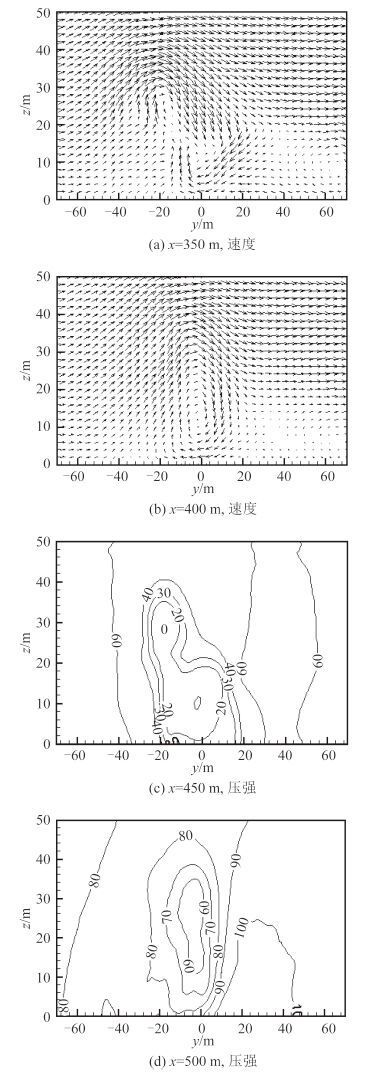图 6 航母后方垂直截面上的时均速度和时均压强（Pa）分布 Fig. 6 The time-averaged velocity and pressure distribution of the aircraft carrier rear vertical section
4 结语

1）侧向来风条件下，航母前部甲板上方压强的变化规律基本一致，其时均值、变化幅度与周期差异不大。后部甲板上方压强时均值较前部分有所增加，但是增加不多，压强变化幅度和周期均略有增大。

2）从时均流场看，当来风为侧向风时，在甲板的前半部分，仅在迎风一侧的舰舷处诱导出了 1 个较大的涡，该涡随着离开舰首距离的增加，强度减弱；而在背风一侧舰舷处，没有看到明显的涡流场结构。

3）在甲板的后半部分，除了迎风侧的涡仍然存在外，在舰岛后部又发出了一对对向涡。迎风侧的涡，随着距舰尾距离越近，尺寸有所增加，涡心逐渐向甲板中心线靠近；而舰岛后的对涡则逐渐离开舰体成为脱体涡，舰舷附近流动成为平缓的水平流。

4）脱体涡离开航母舰体后，逐渐合并成了一个大涡，高度上涡心向海面附近移动，在水平方向上则逐渐向风下游移动。在该涡的背风侧存在着一个很大的下洗速度区域，远高于甲板，而且其速度绝对值也不小。

5）尾流中靠近航母的横向垂直截面上存在着 2 个位于首尾对称面附近的同向大涡，1 个涡心高于甲板，另一个涡心则低于甲板，较高涡的迎风侧为上升气流，背风侧为下洗气流。在远离航母的截面上合并为一个涡。

  ﻿HEALEY J V. The Aerodynamics of Ship Superstructures[C]//.In: Proceedings of AGARD Conference-Aircraft Ship Operations held in France, AGARD-CP-509, 1991: 4.1-4.14.  POLSKY S, NAYLOR S. CVN airwake modeling and integration: initial steps in the creation and implementation of a virtual burble for F-18 carrier landing simulations[C]//. AIAA 2005-6298, AIAA Modeling and Simulation Technologies Conference and Exhibit, San Francisco, California, August, 2005: 15-18.  FINDLAY D B, GHEE T. Experimental investigation of ship airwake flow control for a US navy flight II-A class destroyer (DDG)[C]//. AIAA 2006-3501, 3rd AIAA Flow Control Conference, San Francisco, California, 2006: 5-8.  BOGSTAD M C, HABASHI W G, AKEL I, et al. Computational-Fluid-Dynamics Based Advanced Ship-Airwake Database for Helicopter Flight Simulators[J]. Journal of aircraft , 2002, 39 (5) :830–838. DOI: 10.2514/2.3003  BRIDGES D O, HORN J F, ALPMAN E, et al. Coupled flight dynamics and CFD analysis of pilot workload in ship airwakes[C]//. AIAA 2007-6485, AIAA Atmospheric Flight Mechanics Conference and Exhibit, Hilton Head, South Carolina, August, 2007, 20-23.  REHM R G, BAUM H R. The Equations of Motion for Thermally Driven, Buoyant Flows[J]. Journal of Research of the NBS , 1978, 83 :297–308.  DEARDORFF J W. Numerical Investigation of Neutral and Unstable Planetary Boundary Layers[J]. Journal of Atmospheric Sciences , 1972, 29 :91–115. DOI: 10.1175/1520-0469(1972)029<0091:NIONAU>2.0.CO;2  DEARDORFF J W. Stratocumulus-capped mixed layers derived from a three-dimensional model[J]. Boundary-Layer Meteorol , 1980, 18 :495–527. DOI: 10.1007/BF00119502  WERNER H, WENGLE H. Large-eddy simulation of turbulent flow over and around a cube in a plate channel[C]. In 8th Symposium on Turbulent Shear Flows, 1991, 34: 155-168.  FLOYD J, FORNEY G, HORTIKKA S, et al. Fire Dynamics Simulator(Version 6)-Technical Reference Guide[M]. NIST Special Publication 1018, 2013.  FLOYD J, FORNEY G, HOSTIKKA S, et al. Fire dynamics simulator(version 6)-user's guide[M]. NIST Special Publication 1019, 2013.